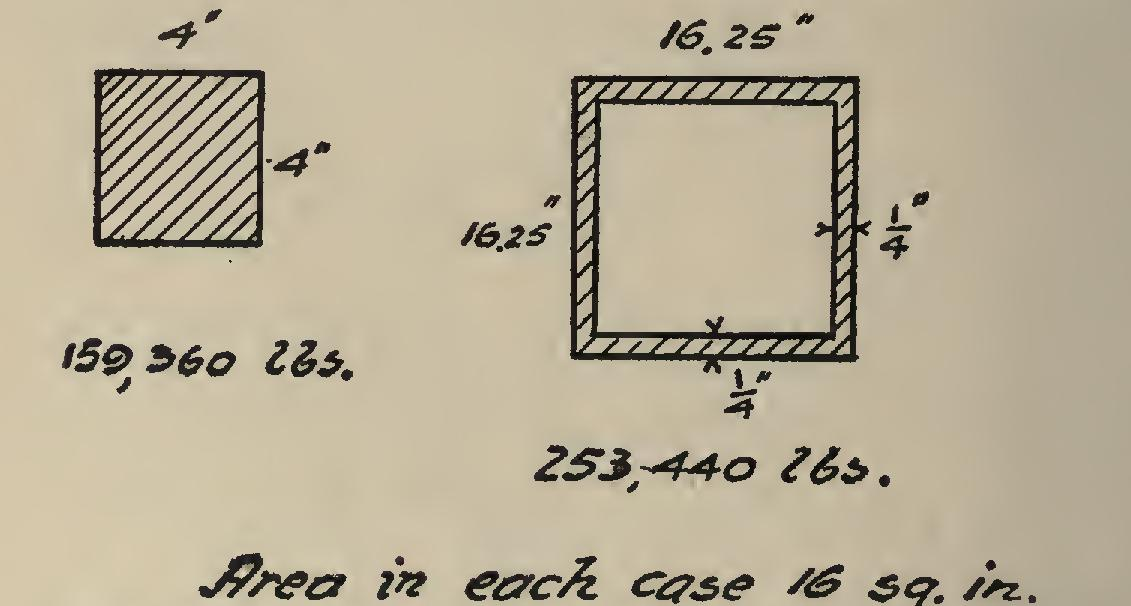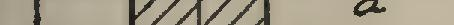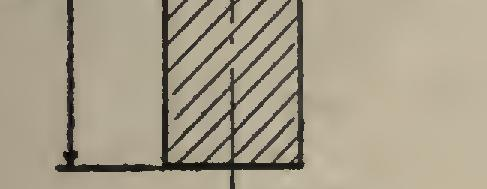Columns 46

column, axis, gyration, radius, fig and single

Page: 1 2

COLUMNS 46. Strength of Columns. The strength of a column depends not only on its length and on the area of its cross-section, but also on the shape and position of the material in the column. The writer took a sheet of the paper on which this book is printed, and, holding it out straight, pressed down with it upon a scale. The scale registered pound when the paper crumpled. Another sheet was taken, and rolled into a tube Fig. 66. Showing Distribution of Areas in Compression Members.% inch in diameter. The scale registered pounds before the paper crumpled. Again, sup pose that a column 12 feet long, consisting of a solid bar 4 by 4 inches=16 square inches in area, were used. The strength would be 159,360 pounds if designed according to the formula 17,100-57 l/r given in (C 141). Now, if the same amount of material were put into a column with cross-section by inches, and the sides were inch thick, the column would hold 253, 440 pounds, an increase in strength of 94,080 pounds, or nearly two-thirds of the original load. From this it will be seen that the farther away from the center of the column the material is lo cated, the stronger is the column. Fig. 66 shows the two sections.

Radius of Gyration. In picking out a col umn, it is important, for any given shape, to know how the area lies in regard to the distance from its center. This is given for different shapes, and is indicated by the radius of gyra tion.

a.Y? =1.,5 rig. 67. rig. 68.

It is important that the column be symmet rical in section. Any departure from this makes the column weaker in one direction than the other. If a board 6 inches wide and 2 inches thick has a load put upon it, it will break on the broad side—that is, by bending about an axis parallel to the broad side. If the radius of gyration were computed for the axis a-a (Fig. 67) it would be found equal to 1.74. If it were computed for the axis b-b, it would be found equal to 0.58. This shows that the ma terial is placed farther from the axis a-a than from the axis b-b. It also shows that the section

is many times stronger about the axis a-a than about the other; and if it were supported on the sides so that it could not bend except about the r, Po r eo',/ieh,\$eov,o/ Po.* Fig. 69. Radius of Gyration in Column with Knee-Brace.axis a-a, the column would hold a load several times what it would if it were left free to bend about the axis b-b.

For the reason that they are unsymmetrical, single shapes such as channels, I-beams, etc., make very inefficient columns, as they are much weaker in one direction than in the other. Take an 18-inch 55-pound I-beam, for example. In (C 97-98), columns 9 and 10, are found the radii of gyration r and r' given as 7.07 and 1.15. This shows (Fig. 68) that the I-beam is much stronger about the axis than about the axis b-b. Also, a comparison of I-beams of any given depth will show that the lighter I-beams are the most eco nomical, since they have a greater radius of gyration than the heavier ones, thus showing that more strength per pound of metal is ob tained from the lighter than from the heavier. For example, the 15-inch 42-pound I-beam gives r=5.95, or 5.95-÷-42=0.142 for each pound; while the 15-inch 100-pound gives r=5.53, or 5.53-F100=0.055 for each pound, showing that the lighter beam is about times more economical.

An investigation of columns 9 and 10 (C 101 102) will show that the single channels are even more uneconomical than single I-beams. Col umns like Fig. 65, j, are open to the same objec tion as single shapes. If, however, a column has a brace or some other member exerting a force against one side of it, and the smallest radius of gyration r' is sufficient for the vertical load, then column sections of single shapes are of use, since a larger radius of gyration r will be required to make the columns safe against the action of the thrust. The case of the column of a mill building where a knee-brace is used, is an example of this kind of loading (see Fig. 69).

Page: 1 2